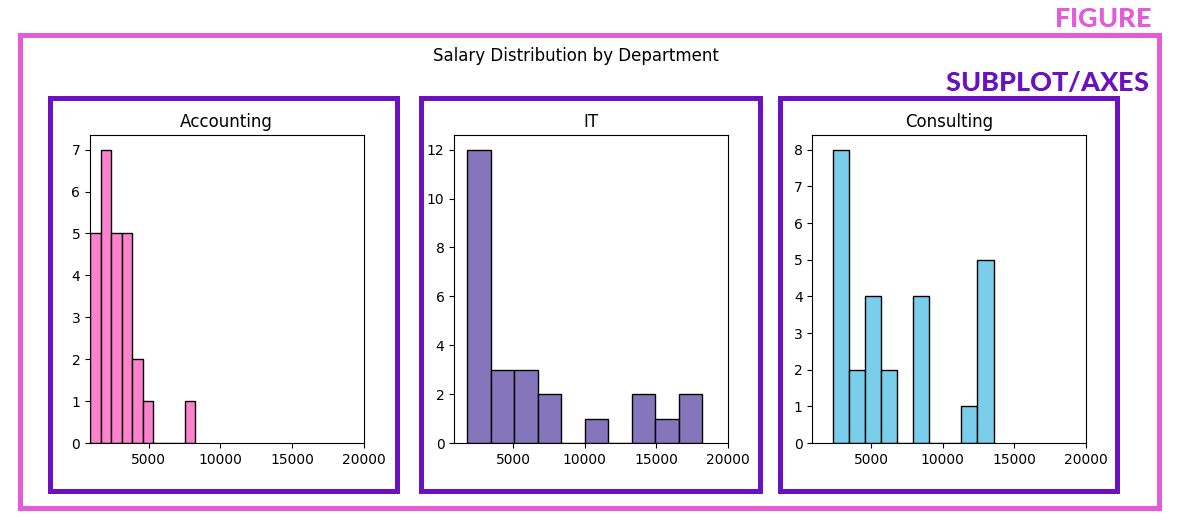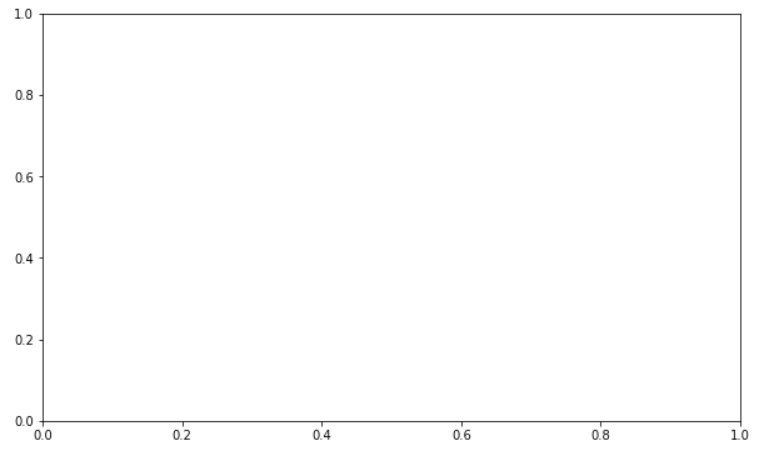Deals Of The Week - hours only!Up to 80% off on all courses and bundles.-Close
Introduction
Line plots
3. Figures and subplots
Multiple histograms
Other plot types
Summary

## Instruction

Great! The picture below presents the line plot that we want to draw using climate data about Barcelona and Toronto (you can check the contents of the dataset using the button on the right). The picture also explains a very important difference between figures and subplots:In matplotlib, a figure refers to the whole surface on which you draw. Think of it as your canvas.

A subplot is basically just a plot: a bar plot, a line plot, a histogram, a pie chart etc. Confusing as it may seem, a subplot can also be called an axes. Oddly enough, it's a singular noun (one axes, two axes). A figure can contain multiple subplots.

To start drawing plots, we need to create at least one figure, with at least one subplot inside it:

figure = plt.figure(figsize=(8, 4))
subplot = plt.subplot()
plt.show()

On line 1, we create a new figure: 8 inches wide, 4 inches high. On line 2, we create a new subplot with default settings. It will be automatically assigned to the figure we have just created. On line 3, we finally show the whole figure.

The result is as follows:## Exercise

figure = plt.figure(figsize=(10, 6))